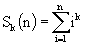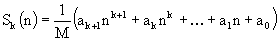Online JudgeProblem SetAuthorsOnline ContestsUser
Web Board
F.A.Qs
Statistical Charts
Problems
Submit Problem
Online Status
Prob.ID:
Register
Authors ranklist
Current Contest
Past Contests
Scheduled Contests
Award Contest
Register
Language:
Sum of powers
 Time Limit: 1000MS Memory Limit: 10000K Total Submissions: 956 Accepted: 459

Description

A young schoolboy would like to calculate the sumfor some fixed natural k and different natural n. He observed that calculating ik for all i (1<=i<=n) and summing up results is a too slow way to do it, because the number of required arithmetical operations increases as n increases. Fortunately, there is another method which takes only a constant number of operations regardless of n. It is possible to show that the sum Sk(n) is equal to some polynomial of degree k+1 in the variable n with rational coefficients, i.e.,We require that integer M be positive and as small as possible. Under this condition the entire set of such numbers (i.e. M, ak+1, ak, ... , a1, a0) will be unique for the given k. You have to write a program to find such set of coefficients to help the schoolboy make his calculations quicker.

Input

The input file contains a single integer k (1<=k<=20).

Output

Write integer numbers M, ak+1, ak, ... , a1, a0 to the output file in the given order. Numbers should be separated by one space. Remember that you should write the answer with the smallest positive M possible.

Sample Input

`2`

Sample Output

```6 2 3 1 0
```

Source

[Submit]   [Go Back]   [Status]   [Discuss]Home PageGo BackTo top